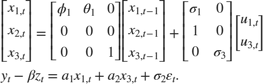# Implicitly Create Diffuse State-Space Model Containing Regression Component

This example shows how to implicitly create a diffuse state-space model that contains a regression component in the observation equation. The state model contains an ARMA(1,1) state and random walk.

Write a function that specifies how the parameters in params map to the state-space model matrices, to the initial state values, and to the type of state. Specify the regression component by deflating the observations within the function. Symbolically, the model is:% Copyright 2015 The MathWorks, Inc. function [A,B,C,D,Mean0,Cov0,StateType,DeflateY] = diffuseRegressionParamMap(params,y,z) % Diffuse state-space model with a regression component parameter mapping % function example. This function maps the vector params to the state-space % matrices (A, B, C, and D) and indicates the type of states (StateType). % The state model contains an ARMA(1,1) model and a random walk. varu1 = exp(params(3)); % Positive variance constraint vare1 = exp(params(5)); A = [params(1) params(2); 0 0]; B = [sqrt(varu1) 0; 1 0]; C = [varu1 0]; D = sqrt(vare1); Mean0 = []; % Let software infer Mean0 Cov0 = []; % Let software infer Cov0 StateType = [0 0 2]; DeflateY = y - params(6)*z; end 

Save this code as a file named diffuseRegressionParamMap.m to a folder on your MATLAB® path.

Create the diffuse state-space model by passing diffuseRegressionParamMap as a function handle to dssm.

Mdl = dssm(@(params)diffuseRegressionParamMap(params,y,z)); 

dssm implicitly creates the diffuse state-space model. Usually, you cannot verify implicitly defined state-space models.

Before creating the model, ensure that the variables y and z exist in your workspace.